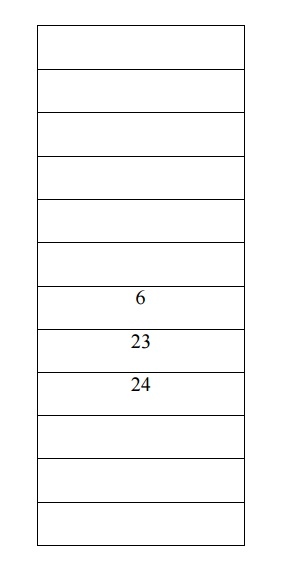Home | | Programming and Data Structures I | Re Hashing Collision

# Re Hashing Collision

Collision occurs when a hash value of a record being inserted hashes to an address that already contain a different record(i.e) when two key values hash to the same position.

RE-HASHING Collision:

Collision occurs when a hash value of a record being inserted hashes to an address that already contain a different record(i.e) when two key values hash to the same position.

Collision Resolution:

The process of finding another position for the collide record is said to be collision resolution stategy.

Two categories of Hashing: àopen Hashing

a) Separate Chaining. -Linked list àClosed Hashing

i) Linear Probing. - Hi(X) = (HASH(X) +i)  % tablesize

ii) Quadratic Probing - Hi(X) = (HASH(X) +i2) % tablesize iii)Double hashing. - Hi(X) = (HASH(X) +i* HASH2(X) % tablesize

HASH2(X)=R-(X % R) R is a prime number that is smaller than the table size

If the table gets too full,then the rehashing method builds new table that is a about twice as big and scan down the entier original hashtable ,computing the new hash value for each element and inserting it in the new table .

Rehashing is very expensive operation, the running time is O(N),since there are N element to rehashing and the table size roughly 2N.

Rehashing can be implemented in several ways with quadratic probing such as:

àRehashing,as soon as the table is half full;

àRehashing only when an insertion fails.

àRehashing when the table reaches a certain load factor

Eg:13,15,24,6 ,23are to be inserted in hash table of size 7.The table will be 70% full.

A new table is created as the table is so full. The new hash function is then h(X) =X mod 17